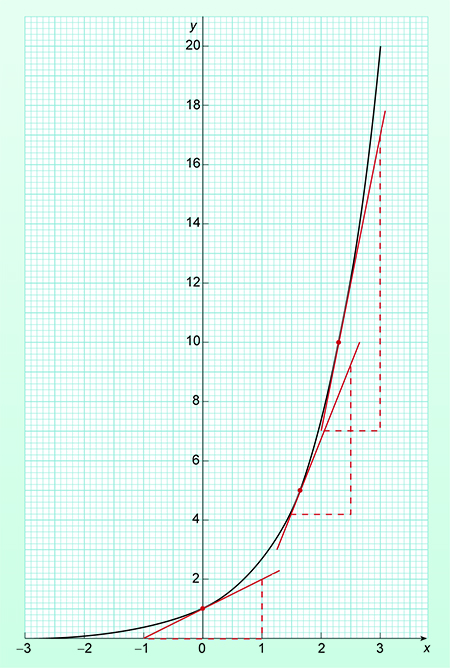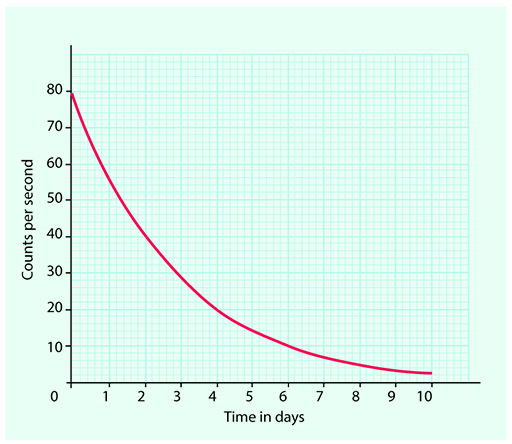Science, Maths & Technology

### Become an OU studentMathematics for science and technology

Start this free course now. Just create an account and sign in. Enrol and complete the course for a free statement of participation or digital badge if available.

# 3 Natural logarithms

The most frequently used bases for logarithms are 10 and the number ‘e’. Rather like , the irrational number ‘e’ occurs frequently in many branches of mathematics and its applications to science and engineering. Logarithms to base 10 are known as common logarithms and those to base ‘e’ are called natural or Napierian logarithms after the mathematician who discovered them.

Natural logarithms have the property that loge ex = x.

Natural logarithms are used to solve equations that contain the exponential function ex where e is the irrational number 2.718281828 correct to ten significant figures. A graph of this function is shown in Figure 2.Figure 2 The exponential function ex

To work with natural logs on a calculator there is usually a button labelled loge or ln, with the inverse ex often accessed by the 2nd function key.

The value of the constant e can easily be found by calculating the value of e1.

An example of an exponential function in science is radioactive decay. The half-life of a radioactive element is a constant value. This means that no matter how long the decay process has been continuing for it always takes the same time for the radioactivity to fall by half. If you compare Figure 2 to Figure 3 you should be able to see that they have similar shapes – although Figure 2 shows growth and Figure 3 decay.When you work with logarithms to base 10 it is convention to drop the subscript and just write log x. Natural logarithms are written as In x. This means that the rules for logarithms, from section 2, can be written as follows for natural logarithms.

## Activity 4 Solve the equations

Solve the equations for x, showing your answers using natural logs. For example, 5 ln 3.

Remember that  ln ex = x.

1. 15 = 3e2x
2. 2ex/10 + 16 = 20

### Discussion

1. 15 = 3e2x

Divide both sides by 3

5 = e2x

So,  ln 5 = 2x  as  ln e = 1

Remember that logaa = 1, so  ln e = 1.

1. Subtract 16 from both sides

Divide both sides by 2

So,

Multiply both sides by –10

The final thing that you need to be able to do with logarithms is change the base.